Contents >> Applied Mathematics >> Numerical Methods >> Ordinary Differential Equations >> Euler’s method

 Ordinary differential equations - Euler's method Euler's method Let's consider the differential equation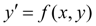(1) with an initial condition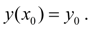Substituting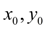into the equation (1), we’ll receive value of a derivative in a point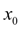: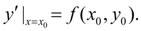At small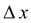the following expression takes place: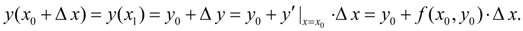Designating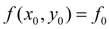, let’s rewrite the last equality in the form of: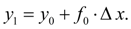(2) Accepting now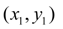for a new initial point, precisely also we’ll receive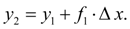In the general case we’ll have: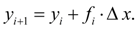(3) It also is Euler's method . The value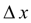refers to as step of integration . Using this method, we receive the approached values у , at as the derivative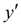actually does not remain to a constant on an interval in length. Therefore we receive a mistake in definition of value of function у , that greater, thanis more. Euler's method is the elementary method of numerical integration of differential equations and systems. Its defects are a small accuracy and a regular accumulation of mistakes. More exact is Euler's modified method with recalculation . Its essence that at the first we find from the formula (3) so-called «a gross approach» (prediction):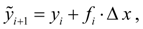and then the recalculation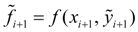gives us too approached, but more exact value (correction):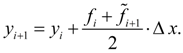(4) Actually the recalculation allows to consider, though approximately, a change of a derivative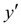on a step of integration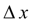as its values in the beginning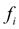and in the end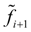of a step (Fig. 1) are considered, and then their average is chosen. Euler's method with recalculation (4) is in essence the 2-nd order Runge-Kutta method . This will become obvious of the further.Fig. 1. The geometric representation of Euler’s method with recalculation.

 < Previous Contents Next >